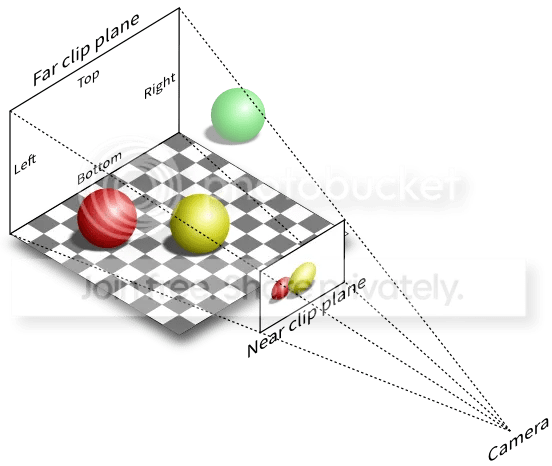Suppose you have an object on the 3D screen, maybe a player. You want to put a 2D (flat) message directly over his head. How do you do it?

Let’s assume we want to put some text on top of the red ball in this scene.OpenGL draws the balls to look as though they are in 3D (in the checkered area of the image), but of course, it actually draws them on your 2D screen (labelled “near clip plane” above). So we want to know where the red ball is actually drawn on your 2D iPad screen.

```function draw()
background(220)
--------------------------- set up 3D
perspective()
camera(0,0,0,0,0,-500)
--------------------------- draw our ball
pushMatrix()
translate(ballPos.x, ballPos.y, ballPos.z)
RedBall:draw() --draw our ball
popMatrix()
--------------------------- go back to 2D to write text
ortho()
viewMatrix(matrix())
text("message text", X, Y)
end
```

The question is – where is X and Y?

The short answer is, we find them like this

```function draw()
background(220)
--------------------------- set up 3D
perspective()
camera(0,0,0,0,0,-500)
--------------------------- draw our ball
pushMatrix()
translate(ballPos.x, ballPos.y, ballPos.z)
RedBall:draw() --draw our ball
local m = modelMatrix()*viewMatrix()*projectionMatrix()
local X, Y = (m/m+1)*WIDTH/2, (m/m+1)*HEIGHT/2
popMatrix()
--------------------------- go back to 2D to write text
ortho()
viewMatrix(matrix())
text("message text", X, Y)
end
```

This code assumes you want the 2D position of the place you have translated to already. But suppose you want the position of the top of your object, so you can draw something above it?

You can get the 2D position of any 3D point p by adding in an extra line, like this, which will adjust the translation part of the matrix.

```local m = modelMatrix()*viewMatrix()*projectionMatrix()
m = m:translate(p.x, p.y, p.z)
local X, Y = (m/m+1)*WIDTH/2, (m/m+1)*HEIGHT/2
```

### How it works

If you don’t care how this works, you can take the code and stop reading.

I’m going to explain as simply as I can (no difficult math) how this works. If you want the full mathematical explanation, you’ll find it here (in sections 8 and 9).

### What are the matrices for?

When you draw something in 3D, you will often want to move it (“translate” it) to a position in your scene, maybe rotate it, then draw it. You then set up the camera in a certain place, pointing in a certain direction.

The three matrices are a clever way of storing the translations and rotations

• modelMatrix converts all the vertex positions of your object [which will usually be centred on (0,0,0)] to their position in your scene, including any rotation
• viewMatrix rotates the whole scene, so the part you want to show is in front of the camera*
• projectionMatrix collapses the z axis so the result is a 2D image. It doesn’t adjust for distance from the camera, but it has the information we need to do that

* which led to a funny joke in Futurama – “The engines don’t move the ship at all. The ship stays where it is, and the engines move the universe around it”.

So by combining these three matrices, we can figure out where a 3D point will end up being drawn on your 2D screen.

First, I’ve recently written a couple of posts explaining how modelMatrix works, and how the translated x,y and z positions are stored in the bottom left of the matrix. This is m, m and m of the matrix m.

Our combined matrix has the translated x,y, z values in the same place. But there is some unfinished business. They need to be adjusted (resized) for their distance from the camera, which is stored in the bottom right of m, in m (it is projectionMatrix that puts this value there). So we need to divide the x and y values by m.

This gives values in the -1 to +1 range, which OpenGL then converts to a position on your screen. We will have to do that ourselves, so we add 1, and multiply by WIDTH/2 or HEIGHT/2, to get the final screen position.

You can see this works by imagining the x value m / m = -1, which should be at the left of the screen. Our formula above is

`(m/m+1)*WIDTH/2 = (-1 + 1)*WIDTH/2 = 0`

which is correct.

Similarly, if m / m = +1, ie at the right of the screen, our formula is

`(m/m+1)*WIDTH/2 = (+1 + 1)*WIDTH/2 = WIDTH * 2/2 = WIDTH`

which is correct.

But even if that’s not very clear, you now have just three lines of code that give you the solution. Enjoy.

And here is some test code that puts a 2D yellow nose on a little astronaut as he zooms around in 3D space.

```function setup()
pos=vec3(0,0,-500)
end

function draw()
background(50)
--make the position vary using "noise"
local e = ElapsedTime
pos=pos+vec3(noise(e+.10),noise(e+.57),noise(e-.16)*3)*5
--set up 3D
perspective()
camera(0,0,0,0,0,-1)
--translate and draw image
pushMatrix()
translate(pos.x,pos.y,pos.z)
sprite(img,0,0) --draw image
--calculate X,Y
local m=modelMatrix()*viewMatrix()*projectionMatrix()
local X,Y=(m/m+1)*WIDTH/2, (m/m+1)*HEIGHT/2
--go back to 2D
popMatrix()
ortho()
viewMatrix(matrix())
fill(color(255,255,0))
ellipse(X,Y,10) --put the yellow nose on the image
end
```

From → 3D, Graphics, Programming